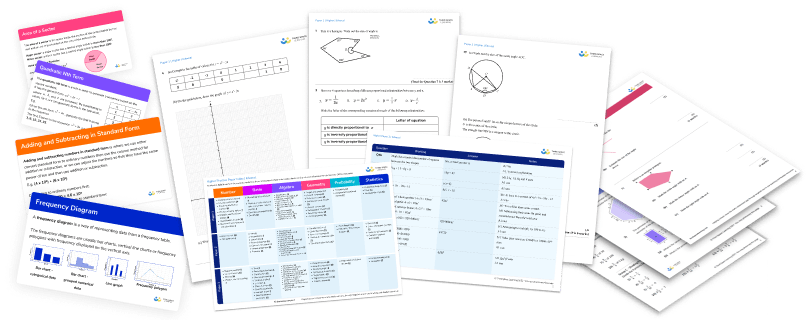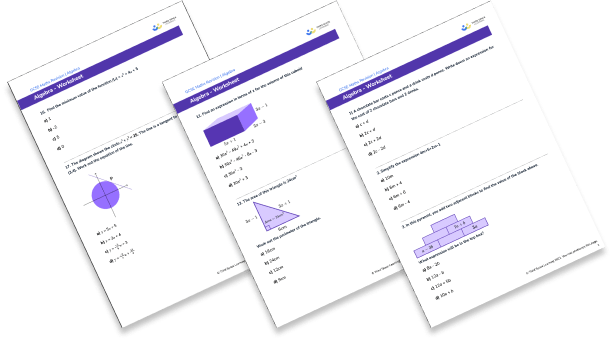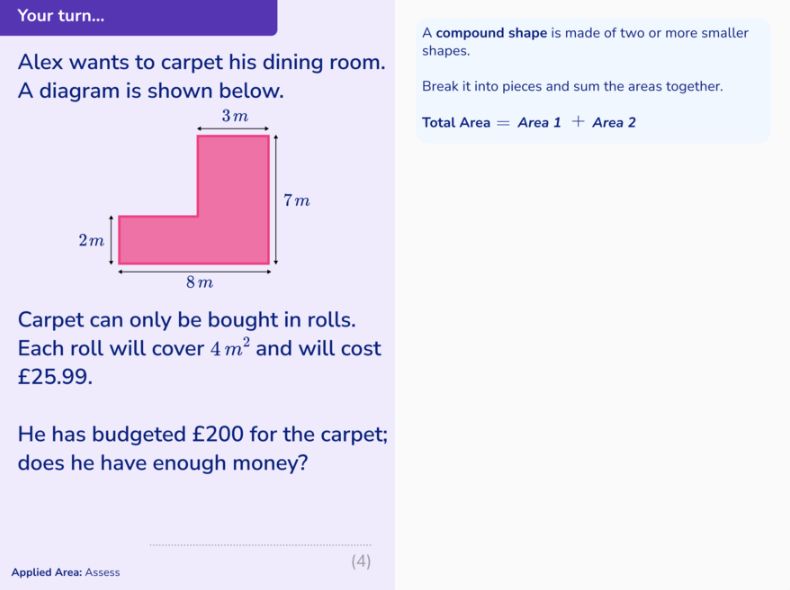Teaching support from the UK’s largest provider of in-school maths tuition.one to one lessonsschools supported

Scalable, affordable online support for KS3 students from maths and pedagogy experts

See it in action

FREE KS3 maths resources

Here you can find all of our free KS3 resources; explore the collections or navigate to each section to filter resources by topicExplore all resources
Key Stage 3

Scalable, affordable online support for KS3 students from maths and pedagogy experts

See it in action

# Year 8 Maths: What Students Learn And How Best To Support Them

By year 8 maths, students are generally settled into secondary school. They have found their feet and know the routines and expectations of the school. This gives them the confidence to show their personalities more, which can lead to some challenging behaviour but it also means that teachers can get to know students and classes more individually and form positive working relationships with them.

Whilst some students begin to lose motivation in year 8, many are still keen to learn. They have developed their basic maths skills and are ready to be challenged with some new ideas. They will really enjoy lessons which are a little bit different – applying their skills to real life situations, playing games and solving problems.

### What will students learn in year 8 maths?

The study of maths can be broken down into a number of sub-categories. Within the National Curriculum, these are:

• Number
• Algebra
• Ratio, proportion and rates of change
• Geometry and measures
• Probability
• Statistics

Alongside this, it is expected that key stage 3 students are given the opportunity to develop their skills in ‘working mathematically’, meaning that they should:

• Develop fluency in the number system, the language of algebra and mathematical terminology.
• Practise their mathematical reasoning; making links between numerical, algebraic and graphical representations, deducing mathematical relationships and constructing mathematical proofs or counter examples.
• Develop their problem solving skills and begin to model problems mathematically.

These skills should be developed and utilised throughout the maths curriculum.

Let’s have a look at what a typical Year 8 maths student might expect to cover within each of the six categories:Download a free worksheet resource, with 15 algebra practise problems for students in KS3 and KS4Download Free Now!

### Year 8 maths curriculum at a glance

Although, unlike KS2 maths there is no prescribed programme of study for Year 8 maths. The following list of topics and questions is a fairly representative sample of what is likely to be taught in Year 8.

#### Number topics

• Fractions, decimals and percentages
• Place value
• Negative numbers
• Factors and multiples
• Indices
• Standard form
• Rounding
•  BIDMAS (order of operations)
##### Example questions
1. Calculate 1 2⁄5 + 3 1⁄2
2. Write 0.4 as a fraction
3. A t-shirt costing £20 is reduced by 30%. Find the sale price.
4. Calculate 45x43
5. Find the highest common factor of 12 and 40
6. Simplify 443
7. Write 4500000 in standard form
8. Round 7.9813 to 2 decimal paces
9. Calculate (3+42)2

#### Algebra topics

• Manipulating algebraic expressions
• Expanding and factorising
• Solving equations
• Using formulae
• Sequences
• Graphs
• Inequalities
##### Example questions
1. Factorise 6x+15
2. Solve the equation 4(2y-3)=28
3. Given that v=u+at, find the value of v when u=2, a=5 and t=4
4. Find the nth term of the sequence 4, 7, 10, 13,…
5. Draw the graph of y=2x+1
6. Write down the possible integer values of x when 5x<10

#### Ratio, proportion and rates of change topics

• Ratio
• Direct proportion
• Conversion rates
##### Example questions
1. Divide £30 in the ratio 2:3
2. To make 10 cakes, a recipe uses 150g flour. How much flour would be required to make 25 cakes?
3. £1=$1.20. Convert £300 to$.

#### Geometry and measure topics

• Area and perimeter
• Surface area and volume
• Angles
• Parallel lines
• Properties of polygons
• Transformations
• Speed/distance/time
• Construction and loci
• Bearings
• Congruence
• Pythagoras Theorem
##### Example questions

1. Find the area of this trapezium:

2. Calculate the volume of a cube with side length 8cm.

3. Calculate the volume of a cube with side length 8cm

4. Work out the size of one angle in an equilateral triangle.

5. Gary travelled 60 miles in 1.5 hours. Find Gary’s average speed.

6. Use a ruler and a compass to construct the perpendicular bisector of this line:

7. The bearing of A from B is 112o. Find the bearing of B from A.

8. Determine whether these triangles are congruent:

9. Find the length of the side labelled x:

#### Probability topics

• Theoretical probability
• Experimental probability
• Sample space diagrams
• Venn diagrams
##### Example questions
1. What is the probability of throwing a 6 when rolling a dice?
2. Two dice are rolled and the totals are added together. Draw a sample space diagram to show the possible results.
3. 40 students are asked whether they like maths or science. 20 say maths and 7 say maths and science. 5 say neither. Draw a Venn diagram to show this information.

#### Statistics topics

• Data collection
• Pie charts
• Scatter diagrams
• Averages
##### Example questions
1. Design three questions to find out about how much fast food people eat.
2. Draw a pie chart to show this data: Football 7, Rugby 8, Neither 3
3. Find the mean of these numbers: 7, 8, 10, 3, 2

### Additional KS3 & KS4 maths questions suitable for year 8

It is worth noting that the exact Year 8 maths scheme of work may vary slightly between schools, as the KS3 national curriculum lists the learning outcomes for all KS3 students rather than for students in a single year group. This gives schools some flexibility to decide when they think it is most appropriate to teach each topic in KS3 maths.

### What students are likely to struggle with in Year 8 maths

As students move into Year 8 maths, a little more is expected from them. They are expected to know the basics of the subject before extending on it. Some of the things that Year 8 maths students might find difficult are discussed below:

#### Times tables and basic number facts

Something that holds a lot of Year 8 maths students back is their inability to recall basic number facts quickly. This can lead to them getting lost during examples or whilst completing work on other topics, as they get bogged down with calculations. As teachers and parents, we should prioritise helping our students feel confident with basic number work. This can include helping them learn the facts, or giving them methods to allow them to work out calculations more easily and in a way that they feel comfortable with. Regular, short practice sessions can make a real difference here.

#### Using algebra

In year 8, algebra is still a relatively new idea to many students. Whilst some students love the logic and methodology associated with algebra, other students can panic when they see an algebra question. To help reassure students that algebra is not a big scary subject that they are unable to access, we can introduce algebra topics in familiar ways. For example, we can introduce solving linear equations using function machines and we can introduce substituting into formulae using word problems.

#### Definitions and technical vocabulary

As students progress through their mathematical studies, they will be expected to understand and use more technical maths vocabulary. Some of the mathematical terms students are expected to know in Year 8 maths are: factor, multiple, prime number, square number, denominator, numerator, solve, simplify, factorise, congruent, similar, mean, median, mode and range. Students might need reminding of these definitions regularly.

#### Problem solving and reasoning

Applying what they have learnt to a question which is phrased in a different way or requires a different way of thinking can be difficult for many students. It is something that becomes easier with practise and is something that should be encouraged whenever possible.

Maths is often seen as a number of distinct topics when, in reality, they are all linked. When using maths in real life we rarely use one skill at a time. For example, when cooking we might want to scale a recipe. We do this using proportion. It may require multiplying by a decimal and converting between metric units at the same time. Students often struggle to make links between topics and may struggle with a skill they have previously mastered when applying it to another area of maths.Example of a lesson slide from Third Space Learning’s online1-to-1 tuition encouraging real world application of maths.

### What students can do to help themselves with Year 8 maths

Throughout secondary school, students often find it difficult to help themselves in maths. We need to teach them how they can work independently and guide them to appropriate resources for self study.

Our students are lucky to have a wealth of information and resources at their fingertips. However, this can be overwhelming. It can be difficult for students to choose a suitable book or website that will offer them easy to understand explanations and practice materials. If we can direct students to 2 or 3 quality sources, we can enable them to help themselves.

Learning maths is a process which includes the following steps:

Given that this is how students learn, we need to select resources which offer them these opportunities.

At Third Space Learning, lessons providing detailed explanations and examples along with GCSE maths worksheets specifically designed to increase students skill and understanding of a topic can be found in the GCSE maths resources section.

For those students who need additional support, Third Space Learning offers online one-to-one tuition from professional maths tutors focused on the needs of each child.

Students should be encouraged to use these sources to help them with their homework or if there is an aspect of their classwork that they don’t understand.

### How adults can support children with Year 8 maths

#### Normalise maths

As adults, it is important that we normalise maths for children. This can be done by talking about the maths we are using when we are doing a task such as cooking, planning a trip or measuring something. Children often don’t see maths as something that is used in real life and this can lead to a lack of motivation. Within the maths classroom, real life questions and scenarios can be built into lessons regularly to allow students to see the wide variety of applications that maths has.

#### Practise basic number facts with your children

As children get older, many become resistant to practising their times tables or other number facts with their parents. You can, however, sneak number practice in without making it obvious, home learning doesn’t always mean reciting times tables! You could, for example, play card games or board games, ask them to work out the cost of your shopping list or plan the schedule and budget for a trip.

There are also many fun apps and games available on tablets and phones that children might enjoy using and which may also help with Year 8 maths. You know your child, so make sure that you choose tasks that are appropriate for their level and that they will find enjoyable.

Within the Year 8 maths classroom, number facts should be practised regularly and in a way that allows students to build their skill level and confidence. This will vary for each class and may involve practising limited number skills at a time and introducing new skills gradually.

Read more: KS3 Maths Games will give you plenty of ideas for mathematical games.

A very effective way of learning and retaining information is to teach it to someone else. Ask your child what they have been learning in maths and suggest that you have forgotten how to do it. Let them play the expert and even test you on what you have learnt.

Remember though, do not tell them you were ‘rubbish at maths’ or ‘hated maths at school’ as this only leads to a belief that struggling with maths is normal and accepted. Instead we want them to feel motivated and to believe that we can all improve and learn with the right encouragement and support. Within a maths class, students could teach each other or design a worksheet for a partner. Designing questions on a topic requires a good understanding of that topic.

#### Familiarise yourself with the resources available for your children

If your child’s school has a subscription to a certain site or recommends a certain site or book, familiarise yourself with it. This way, you can help them with any difficulty they may have navigating the site. You can also work through the resources and tasks together.

Within maths lessons, students should be given the opportunity to use these sites with support from a teacher to ensure they are confident with them. Details of the school’s sites/recommended resources can be shared with parents, and parents should be encouraged to look at them.

As parents and teachers, our common aim is for our children to feel confident and motivated in their mathematical studies. Hopefully this post has given you some useful ideas and insights into what your children will be learning and how we can all work together to best support them.

If there is a particular topic or area of study that you would like more ideas or support with, check out the GCSE maths revision pages from Third Space Learning, many of which are also relevant and useful to Year 8 maths students.

Looking for additional support and resources?

You are welcome to download any of the secondary maths resources from Third Space Learning’s resource library for free. There is a section devoted to GCSE maths revision with plenty of maths worksheets and GCSE maths questions. There are also maths tests for KS3, including a Year 7 maths test, a Year 8 maths test and a Year 9 maths test

Other valuable maths practice and ideas particularly around reasoning and problem solving at secondary can be found in our KS3 and KS4 maths blog articles. Try these fun maths problems for KS2 and KS3, KS3 maths games and 30 problem solving maths questions.

For children who need more support, our maths intervention programmes for KS3 achieve outstanding results through a personalised one to one tuition approach.

### What do year 8 children learn in maths?

Year 8 children cover 6 different areas in year 8 maths: Number, Algebra, Ratio and proportion, Geometry, Probability and Statistics. They will build on the work that they have done in year 7 as well as being introduced to some new concepts. These might include multiplying and dividing fractions, standard form, rearranging formulae, probability trees and averages from a frequency table. The exact scheme of work for each year group is likely to vary from school to school.

### What is the difference between KS3 maths and KS2 maths?

Much of the work that children do in KS3 maths builds upon what they have covered in KS2. For example, in KS2 students will spend a lot of time learning about the number system including basic number facts, place value, fractions, decimals and percentages and negative numbers. These skills are all revisited many times and built upon throughout KS3. The ideas and concepts learnt about in KS2 form the building blocks for KS3 and KS4 maths.

Students are also introduced to a number of new concepts and some new mathematical terminology. Students are expected to become more independent with their work and the pace at which they cover content is likely to become quicker.

There is a focus in KS3 on developing students’ mathematical reasoning and problem solving. Students are challenged to apply their knowledge to increasingly difficult problems and to develop their ability to reason and form arguments. These skills are particularly important to students as they move on to their GCSEs and throughout life.

Do you have students who need extra support in maths?
Every week Third Space Learning’s maths specialist tutors support thousands of students across hundreds of schools with weekly online 1-to-1 lessons and maths interventions designed to plug gaps and boost progress.

Since 2013 we’ve helped over 100,000 primary and secondary students become more confident, able mathematicians. Find out more about our GCSE Maths Revision Programme or request a personalised quote for your school to speak to us about your school’s needs and how we can help.

Our online tuition for maths programme provides every child with their own professional one to one maths tutor##### Beki Christian
Maths Teacher and Author
Beki has 11 years experience teaching secondary Maths. She is currently working as a Maths tutor as well as writing resources and blog posts for Third Space Learning.

Related Articles

xDownload a free worksheet resource, with 15 algebra practise problems for students in KS3 and KS4